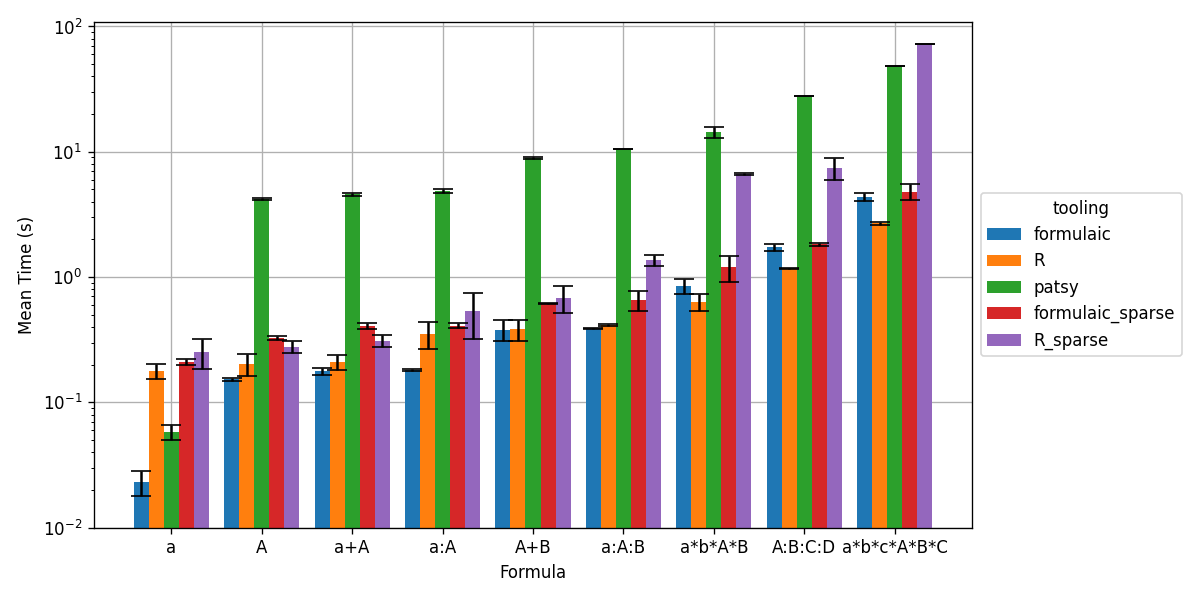An implementation of Wilkinson formulas.

#Formulaic is a high-performance implementation of Wilkinson formulas for Python.

Note: This project, while largely complete, is still a work in progress, and the API is subject to change between major versions (0.<major>.<minor>).

It provides:

• high-performance dataframe to model-matrix conversions.
• support for reusing the encoding choices made during conversion of one data-set on other datasets.
• extensible formula parsing.
• extensible data input/output plugins, with implementations for:
• input:
• pandas.DataFrame
• pyarrow.Table
• output:
• pandas.DataFrame
• numpy.ndarray
• scipy.sparse.CSCMatrix
• support for symbolic differentiation of formulas (and hence model matrices).

## Example code

import pandas
from formulaic import Formula

df = pandas.DataFrame({
'y': [0,1,2],
'x': ['A', 'B', 'C'],
'z': [0.3, 0.1, 0.2],
})

y, X = Formula('y ~ x + z').get_model_matrix(df)


y =

y
0 0
1 1
2 2

X =

Intercept x[T.B] x[T.C] z
0 1.0 0 0 0.3
1 1.0 1 0 0.1
2 1.0 0 1 0.2

## Benchmarks

Formulaic typically outperforms R for both dense and sparse model matrices, and vastly outperforms patsy (the existing implementation for Python) for dense matrices (patsy does not support sparse model matrix output).For more details, see here.

## Related projects and prior art

• Patsy: a prior implementation of Wilkinson formulas for Python, which is widely used (e.g. in statsmodels). It has fantastic documentation (which helped bootstrap this project), and a rich array of features.
• StatsModels.jl @formula: The implementation of Wilkinson formulas for Julia.
• R Formulas: The implementation of Wilkinson formulas for R, which is thoroughly introduced here. [R itself is an implementation of S, in which formulas were first made popular].
• The work that started it all: Wilkinson, G. N., and C. E. Rogers. Symbolic description of factorial models for analysis of variance. J. Royal Statistics Society 22, pp. 392–399, 1973.

## Project details

This version0.6.40.6.30.6.20.6.10.6.00.5.20.5.10.5.00.4.00.3.40.3.30.3.20.3.10.3.00.2.40.2.30.2.20.2.10.2.00.1.20.1.10.0.1

Uploaded source
Uploaded py3